# Body weight in physics ℹ️ definition, formula, how it is measured, how it differs from mass, what the force of weight depends on and what causes it## Concept and definitions

Mass (denoted by the letter m) is one of the physical quantities, such as volume, that determine the amount of matter in an object. There are several phenomena that make it possible to evaluate it. There is an opinion among theorists that some of these phenomena may be independent of each other, but in the course of experiments, no differences were found in the results from the method of mass measurements:

• Inertial. It is determined by the body's resistance to acceleration by force.
• Active and passive gravitational. It is measured by the force of interaction of the gravitational fields of objects.A person feels their mass in contact with another surface. ... It can be a chair, the earth's firmament, an astronaut's chair while accelerating in a rocket. In these examples, we are talking about a quantity that physicists call weight, and subjectively perceived as an apparent weight.

It is equal to the actual measured mass in almost all domestic cases, with the following exceptions:

• The body receives acceleration with a vertical component in relation to the ground. For example, in an elevator or an airplane.
• In addition to the gravity of the Earth, other forces act on the body - centrifugal, gravitational of another from the body, Archimedean.

### Gravitational approach

In most cases, when defining the concept of weight (the accepted designation is P, in Latin it is written as pondus), the so-called gravitational definition is used. In physics textbooks, the weight formula for a body describes a quantity as a force acting on an object as a result of gravity. In the language of mathematics, this is defined by the expression P = mg where: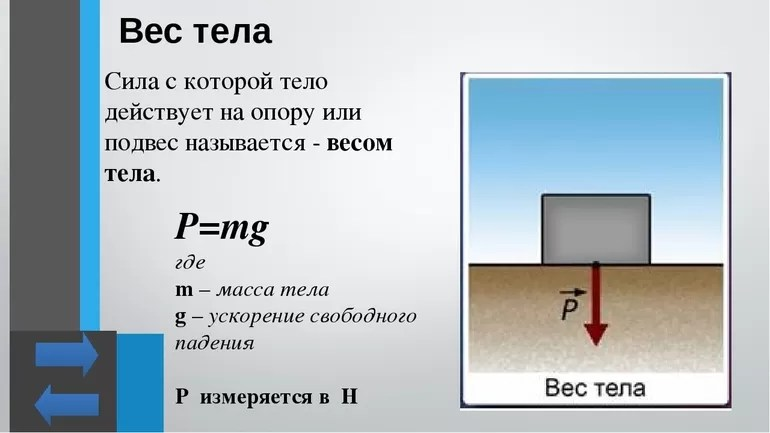• m is the mass;
• g - gravitational acceleration.

The formula implies what the weight is measured in: quantitatively it is calculated in the same units as the force. Therefore, according to the International System of Units (SI), P is measured in Newtons.

The Earth's gravitational field is not uniform and varies within 0.5% over the planet's surface. Accordingly, the value of g is also unstable. The generally accepted value is called the standard and equal to 9.80665 m / s2. In various places on the surface of the Earth, the actual gravitational acceleration is (m / s2):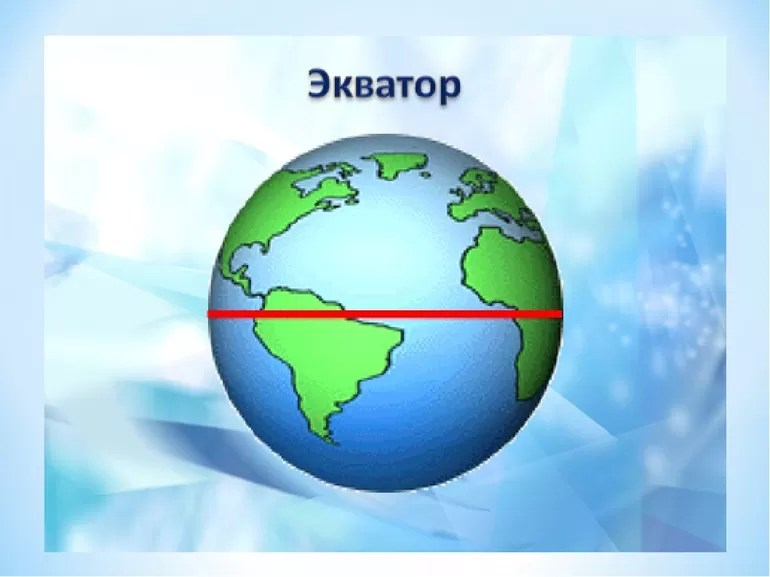• equator - 9.7803;
• Sydney - 9.7968;
• Moscow - 9.8155;
• North Pole - 9.8322.

In 1901, the third General Conference on Weights and Measures established: weight means quantity of the same nature as force, That is, it defined it as a vector, since force is a vector quantity. Nevertheless, some school physics textbooks still take P for a scalar.

### Contact definition

Another approach describes the phenomenon from the standpoint of understanding what force is called body weight. In this case, P is determined by the weighing procedure and means the force with which the object acts on the support. This approach assumes different results depending on the details.For example, an object in free fall has little effect on the support, however, being in zero gravity does not change its weight in accordance with gravitational definition. Consequently, such an approach requires finding the investigated body at rest, under the action of standard gravity, without the influence of the centrifugal force of the Earth's rotation.

In addition, contact detection does not exclude buoyancy distortion, which reduces the measured weight of the object. In air, bodies are also affected by a force similar to that which is immersed in water. For objects with a low density, the effect of the influence becomes more noticeable. An example of this is a negative-weight helium-filled balloon. In a general sense, any action has a distorting effect on the contact weight, for example:• Centrifugal force. As the Earth rotates, objects on the surface are subject to centrifugal forces more pronounced toward the equator.
• The gravitational influence of other astronomical bodies. The sun and moon attract objects on the earth's surface to varying degrees, depending on the distance. This influence is insignificant at the household level, but is noticeably reflected in such phenomena as the ebb and flow of the sea.
• Magnetism. Strong magnetic fields can cause some affected objects to levitate.

## History of the conceptThe concepts of gravity and lightness as inherent properties of physical bodies mentioned by ancient Greek philosophers ... Plato described weight as the natural tendency of objects to find their own kind. For Aristotle, lightness was a property in restoring the order of the basic elements: air, earth, fire and water. Archimedes viewed weight as the opposite of buoyancy. The first contact definition was given by Euclid, describing quantity as the lightness of one thing compared to another, as measured by balance.

When medieval scientists discovered that in practice, the speed of a falling object increased over time. They changed the concept of weight to preserve causal relationships between phenomena. The concept was divided for bodies at rest and those in gravitational fall.

Significant results in theory were achieved by Galileo, who came to the conclusion that the quantity is proportional to the amount of matter in the object, and not to the speed of its movement, as suggested by Aristotle's physics. Newton's discovery of the law of universal gravitation led to the fundamental separation of weight from the fundamental property of objects associated with inertia. The scientist considered environmental factors and buoyancy to be a distortion of the measurement conditions. For such circumstances, he coined the term apparent weight.In the 20th century, Newtonian concepts of absolute time and space were challenged by the work of Einstein. Theory of relativity put all the observers, moving and accelerating, in different conditions. This has led to ambiguity as to what exactly is meant by mass, which, together with gravitational force, has become an essentially frame-dependent quantity.

The ambiguities generated by relativity have led to serious debate in the teaching community about how to define student weights and what should be called them. The choice began to lie between understanding it as a force caused by the gravity of the Earth, and the contact definition arising from the act of weighing.

## Differences with mass

Confusion in understanding how mass differs from weight is inherent for people who do not study physics in detail. There is a simple explanation for this - as a rule, these terms are used interchangeably in everyday life. In the general case, if the body is on the surface of the earth and is motionless, the mass value will be equal to the scalar of the weight in kilograms. A table clarifying the difference between the concepts looks like this:

 Weight The weight It is a property of matter. Always constant. Depends on the action of gravity. A material object is never zero. Can be zero under certain conditions. Does not change depending on location. Decreases or increases in different places of the Earth or depending on the height above its surface. It is a scalar. A vector pointing towards the center of the earth or another gravitational center. Can be measured with balance Measured with a spring balance. Typically measured in grams and kilograms. The unit of force and weight is one - Newton (denoted as N)

The main distinguishing property of mass is that for classical dynamics it is a specific invariant quantity for each body. General relativity describes the transition of mass to energy and vice versa.

Usually, the numerical value between m and P on Earth is strictly proportional. At the everyday level, in order to find out the weight of a body with a known mass, it is enough to remember that objects usually weigh in newtons approximately 10 times more than the value of m in kilograms.

## Measurement methods

In fact, weight can be measured as the force of reaction of the support to the mass, appearing at the point of application. The magnitude of the occurrence of this force is equal in value to the desired P. It can be determined using a spring balance. Since the force of gravity that causes the reported deflection on the scale can vary from place to place, the values ​​will also differ. For standardization, this type of meter is always factory calibrated to 9.80665 m / s2 and then re-calibrated where it will be used.

A lever mechanism is used to measure the mass. ... Since any changes in gravity will have the same effect on known and unknown masses, the balance method allows the result to be the same anywhere on Earth. The weighting factors in this case are calibrated and labeled in units of mass, so the balancing lever allows you to find the mass by comparing the effect of attraction on the target object with the effect on the standard.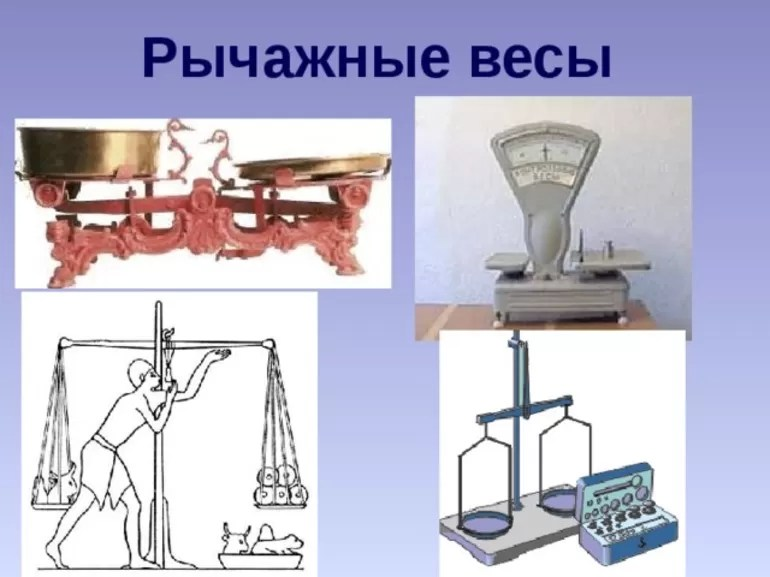In the absence of a gravitational field far from large astronomical bodies, the balance of the lever will not work, but, for example, on the Moon it will show the same values ​​as on Earth. Some of these instruments may be labeled in units of weight, but because they are calibrated at the factory for standard gravity, they will show P for the conditions for which they are set.

This means that the beam balance is not designed to measure the local gravity acting on an object. The exact weight can be determined by calculation by multiplying the mass by the local gravity value from the corresponding tables.

## On other planets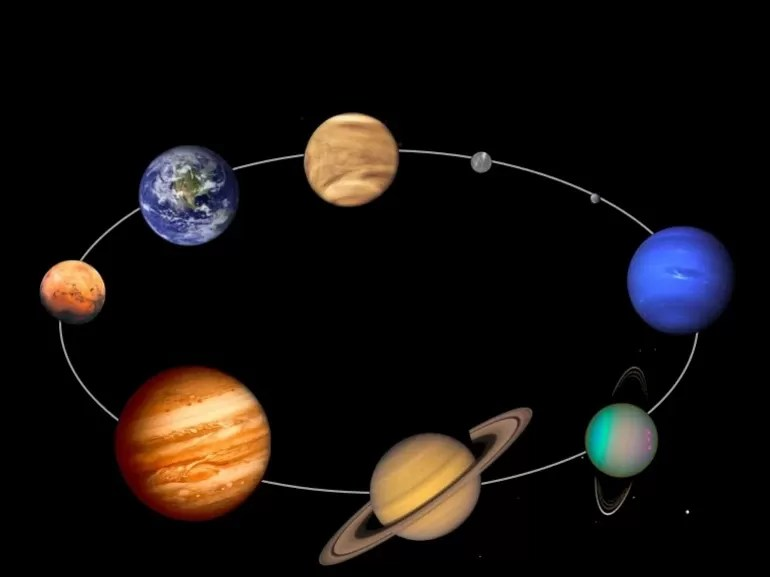Unlike mass, body weight in different places varies depending on the change in the value of gravitational acceleration. The magnitude of the force of gravity on other planets, as well as on Earth, depends not only on their mass, but also on how far the surface is from the center of gravity.

The table below shows the comparative gravitational accelerations on other planets, the Sun and the Moon. The surface for gas giants (Jupiter, Saturn, Uranus and Neptune) means their outer cloud layers, for the Sun - the photosphere. The values ​​in the table do not include centrifugal rotation and reflect the actual gravity observed near the poles.

 Astronomical object How much gravity exceeds Earth Surface acceleration m / s2 The sun 27.9 274.1 Mercury 0.377 3.703 Venus 0.9032 8,872 Earth 1 9.8226 Moon 0.1655 1,625 Mars 0.3895 3.728 Jupiter 2.64 25.93 Saturn 1.139 11.19 Uranus 0.917 9.01 Neptune 1.148 11.28

In order to get your own weight on another planet, you just need to multiply it by the multiplicity number from the corresponding column. The closer to the center of the planet the measurement is made, the higher the value will be, and vice versa. Therefore, despite the fact that the gravitational force of Jupiter due to its huge mass is 316 times higher than that of the Earth, the weight at the level of the clouds, due to their great distance from the center of mass, does not look as impressive as one might expect.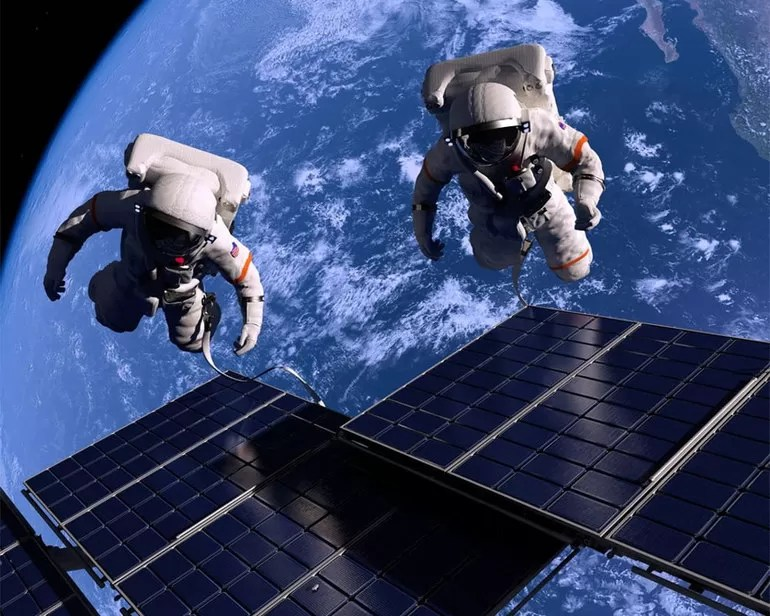Another interesting effect, called weightlessness, is characteristic not only of space. It can be observed under various circumstances and on Earth. For example, in free fall, there is no support to which a force would be applied, which means that the weight will be equal to zero, despite the presence of acceleration of gravity and mass.

A similar phenomenon occurs with the astronauts of the International Space Station in Earth orbit. In fact, it always falls with its inhabitants to the surface of the planet, so its inhabitants are constantly in a state of weightlessness.

Thus, the main rule explaining the observed phenomena and avoiding confusion with mass is as follows: the value of P is always measured using contact weights placed between the object and the reference surface. That is why a body placed on the scales and falling with them will not press on the device, and the scale, accordingly, will show a zero value.There was a German astronomer with the surname Bayer. He developed a system for determining the brightness of the stars and arranged them in accordance with the other Greek alphabet. The brightest began with the letter "alpha", then "beta" and so on. Later, the scientists didn’t change the principle when refining his system, they just distributed the bright stars: "alpha1", 2,3, etc.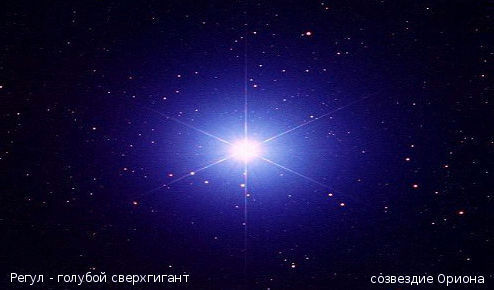bright star of the sky with the designation "alpha1" "Regulus"In physics, thickness, as well as distance (distance) and diameter, are denoted by the letter d.

In the design documentation, designations are used according to GOST 2.321.

The thickness is indicated by the letter s.

The total or overall size according to GOST is indicated by an uppercase letter, which means that the total (overall) thickness should be indicated by the letter S.The perimeter is the sum of the lengths of all sides (or the total length of all the boundaries of the shape). Almost every shape has a different formula for the perimeter, as is the case with area.

In mathematics, as a rule, it is customary to designate the perimeter of a figure by the Latin letter "P".

For example, the perimeter formula looks like this: P = 2 * (a + b).

You can also note the fact that the length of a circle, which is its perimeter (since in the case of a circle, its length is the border of the figure), is denoted not by the letter "P", but by the letter "C" or even l. But this is rather an exception to the rule, in all other cases it is indicated with the letter "P".Speed ​​in physics is a quantitative characteristic of the movement of a body, it is denoted by the letter V. Speed ​​is numerically equal to the path (the path is denoted by S) traversed by the body per unit of time (time is denoted by t).

The unit of measure for speed is meter per second (m / s).Samira Gadzhieva is a famous Dagestan actress and singer, was born in Derbent on June 27, 1991, currently lives and works in Makhachkala, is a Lezginka by nationality.

The growth of Samira Hajiyeva is about 172 centimeters, the artist has personal pages on the social networks Instagram and VKontakte.It's amazing how many people, when using the words "mass" and "weight", do not understand their difference from the point of view of physics and mean the same thing. Meanwhile, this difference is fundamental and huge ...

### Weight

Let's start with the mass. Mass determines the inertial properties of a body. What does this mean? Inertia is the body's ability to resist a change in its state of motion under the influence of force. Try to stop a soccer ball rolling by inertia. And then - a car rolling at the same speed by inertia. In the latter case, it is much more difficult to do this, because the car has a lot of matter. And we can say that the car has more weight. The mass is measured in kilograms, and denoted by the letter m... Body weight is always constant.

### The weight

As far as weight is concerned, it is strength. Like any other force, it is a vector quantity (having a direction of action) and it is measured in newtons ... By definition, weight is the force with which a body acts on a support or suspension:

If a person weighing 70 kg stands motionless on the floor, what forces act on him from the point of view of classical mechanics? Just two. One of them is the force of gravity directed vertically downward. This is the force with which the Earth attracts a person, and it is equal to the product of the person's mass mon the acceleration of gravity g(for the Earth - 9.81 m / s2, let's round this value to 10). Thus, this force will be equal to mg = 70 * 10 = 700H. Often this force is also measured in kilogram-force, kgf ... Its value is equal to the weight of a body weighing 1 kg, therefore, ordinary people often measure weight in kilograms and that is why confusion often arises with weight and mass.

The second force is the reaction force of the support N... A person presses on the floor, and the floor resists it - with exactly the same force as the force of gravity. This force is directed in the opposite direction and is equal in magnitude to the force of gravity. The total force is equal to F = mg-N = 0 .

You may ask - why all this if gravity and weight are one and the same? Nothing of the kind, these are completely different things, they just coincide in this example. Consider an astronaut in a rocket taking off. It is also affected by the force of gravity and the reaction force of the support, but plus to this is added the force that pushes the astronaut upward along with the rocket. In this case, the reaction force of the support Nwill exceed gravity mg , and the astronaut's weight will increase, he will experience overload, although the gravity force and the astronaut's mass have not changed.

In fact, weight is an insignificant term for physicists. From the point of view of physics, it is more correct to call it simply force, and the word "weight" is just a tribute to the linguistic tradition.

Under terrestrial conditions, people usually equate weight and mass, and the scale for all scales is calibrated for terrestrial gravity. However, the interaction of weight and mass is very interesting to observe in conditions other than Earth. So, on the Moon, the force of gravity is 6 times less than that of the Earth, respectively, the weight of the astronaut will also be 6 times less. In this case, its mass will remain unchanged. If we try to hammer a nail into a board on the moon, the hammer will weigh 6 times less. But when hitting the head, it will act on the nail with the same force as on Earth, because the mass of the hammer has not changed.

Bottom line. Mass is an inseparable property of any body. If the sports core mass 7 kg is hard to throw on Earth, it will be just as hard to throw it in zero gravity, despite the fact that it the weight will be zero.

If you liked the article, subscribe to the channel, tell about it on social networks, and we will try not to lose face)